In a previous RPubs document we were playing around with a few examples using data and code from the ggplot2 package and Hadley Wickham’s book, R for Data Science. We were trying to see how we can take different views of the same data set. This time, we will use another type of visual analysis.

It is not difficult to include regression analysis in our charts. Packages in R often make use of the LOESS method – basically a non-linear approach to smooth out the variability in data. We can do this for the entire data set or, as we saw previously, on chosen subsets of the data.

You can find the code on GitHub.

First, we need to load the necessary packages – in this case, dplyr and ggplot2.

library(dplyr)
##
## Attaching package: 'dplyr'
## The following objects are masked from 'package:stats':
##
##     filter, lag
## The following objects are masked from 'package:base':
##
##     intersect, setdiff, setequal, union
library(ggplot2)

In the first graph, we draw the regression line that fits all our data, but we omit the data points. The ggplot function builds a confidence interval (the gray area) around the line as a default.

ggplot(data = mpg) + geom_smooth(mapping = aes(x = displ, y = hwy)) +
ggtitle("Displacement and Highway Mileage") +
labs(x = "engine displacement (in liters)", y = "highway mileage/gallon")
## `geom_smooth()` using method = 'loess'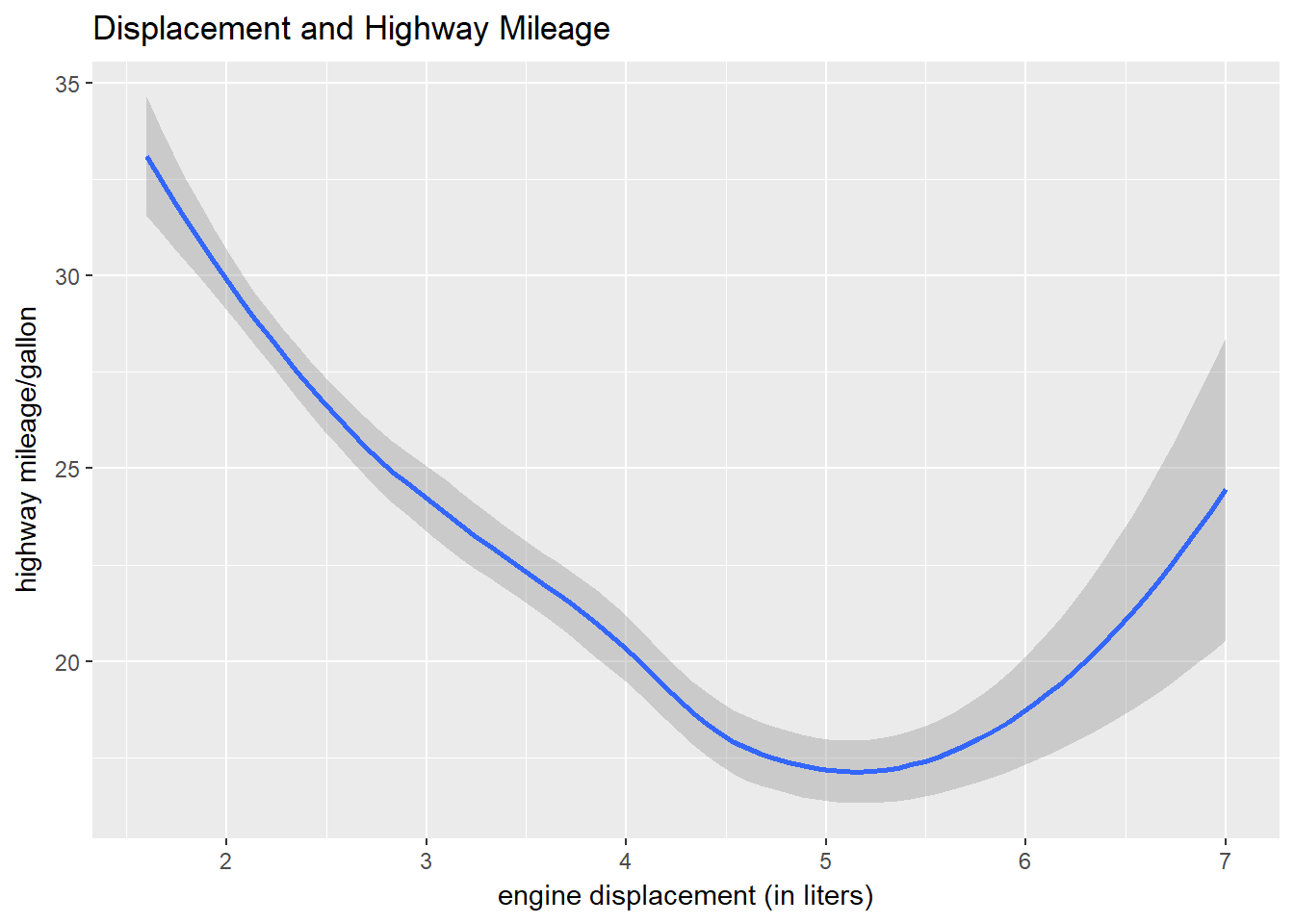It is easy to remove the confidence interval. Just add se = FALSE to the geom_smooth argument. The span argument is used to control the smoothness of the line. Play around with it. You will find the lower the value the more variable the line. But you will reach a limit if you go too low and R will let you hear about it.

ggplot(data = mpg) + geom_smooth(mapping = aes(x = displ, y = hwy), se = FALSE, span = 0.8) +
ggtitle("Displacement and Highway Mileage") +
labs(x = "engine displacement (in liters)", y = "highway mileage/gallon")
## `geom_smooth()` using method = 'loess'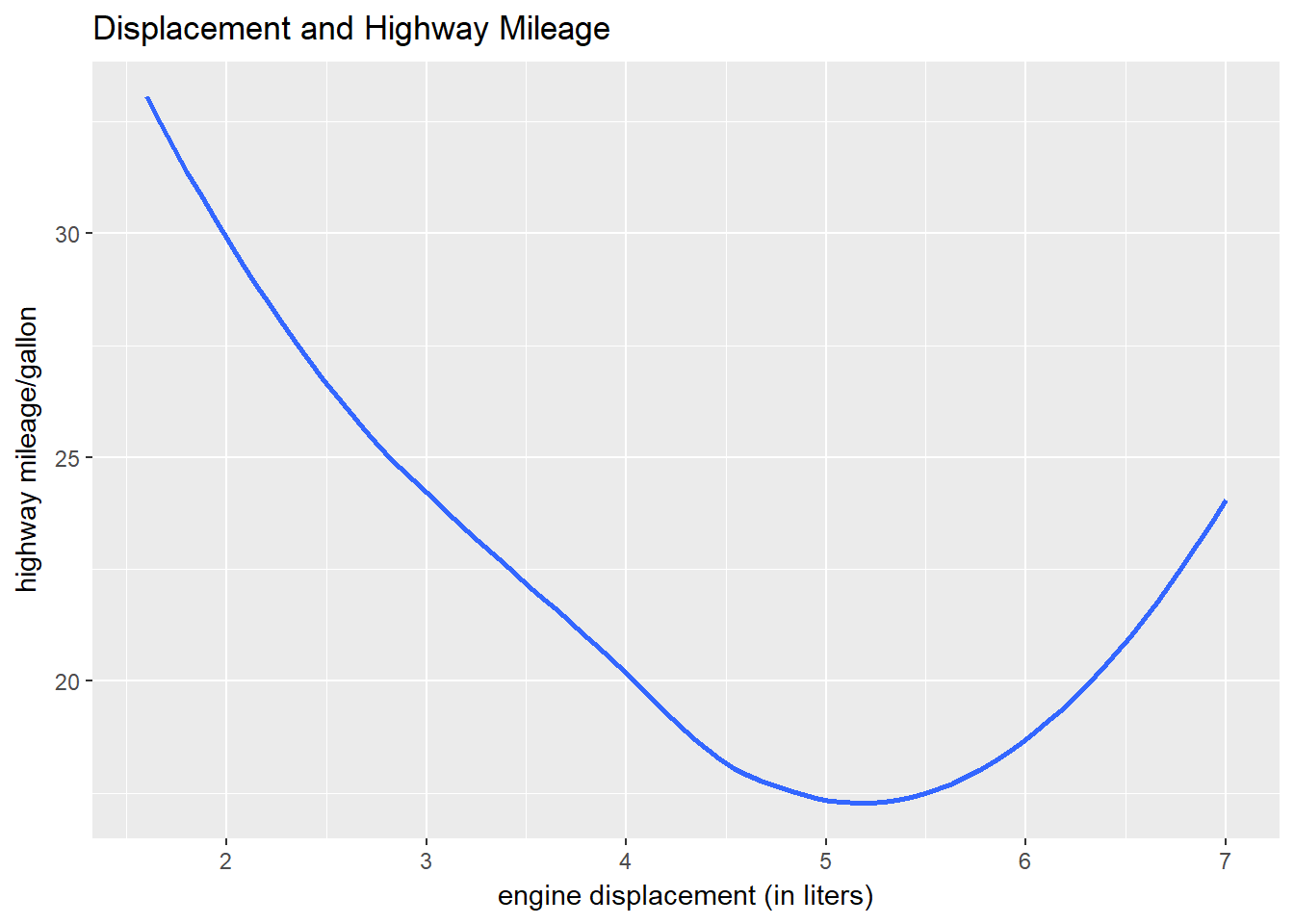Now, we will draw regression lines for different types of data in our data set. Here, we specify linetype = drv to see the regressions for the three drive types included in our data – 4-wheel, front-wheel, and rear-wheel. Each type will be depicted by a different style of line.

ggplot(data = mpg) + geom_smooth(mapping = aes(x = displ, y = hwy, linetype = drv)) +
ggtitle("Displacement and Highway Mileage by Drive Type") +
labs(x = "engine displacement (in liters)", y = "highway mileage/gallon")
## `geom_smooth()` using method = 'loess'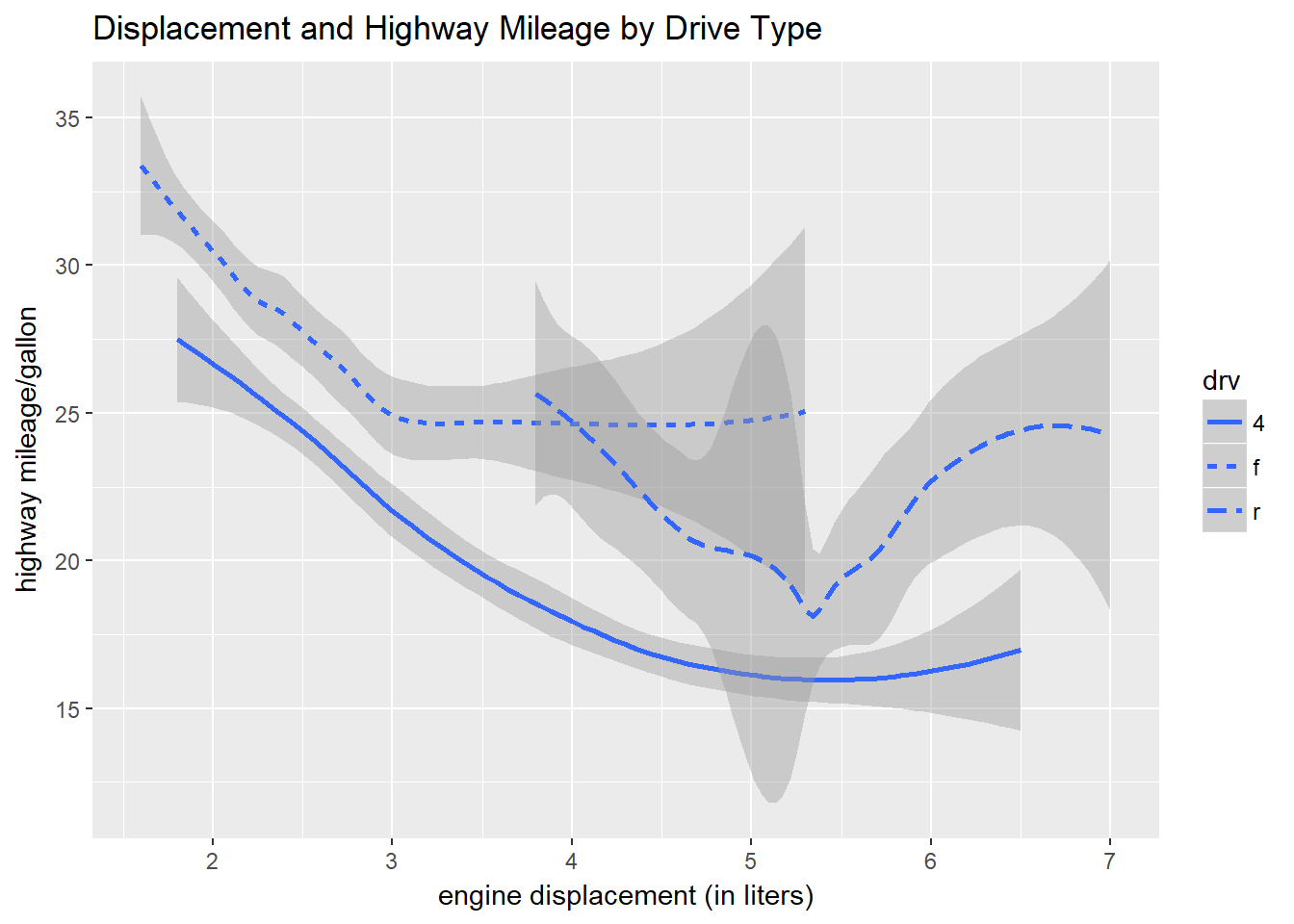We can jazz this up a bit and depict each drive type by a different colored line using col = drv.

ggplot(data = mpg) + geom_smooth(mapping = aes(x = displ, y = hwy, color = drv)) +
ggtitle("Displacement and Highway Mileage by Drive Type") +
labs(x = "engine displacement (in liters)", y = "highway mileage/gallon")
## `geom_smooth()` using method = 'loess'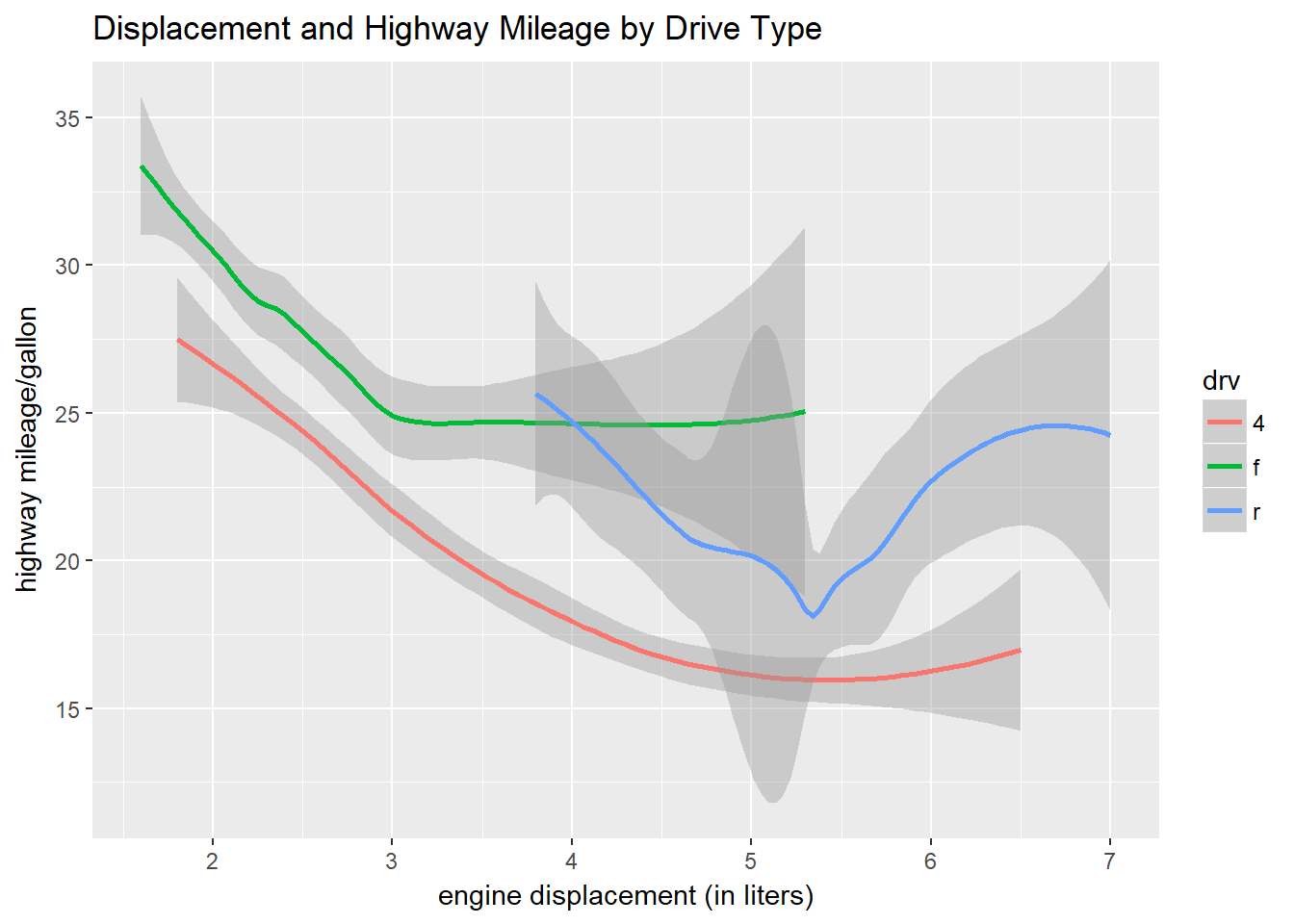Now let’s overlay the data points we are using to draw the regression lines for each drive type. This clutters the graph up a bit, but it adds a new dimension that might be useful for the visual. We can do this by mapping to the aes in the geom_point argument we used previously.

ggplot(data = mpg) +
geom_point(mapping = aes(x = displ, y = hwy, color = drv)) +
geom_smooth(mapping = aes(x = displ, y = hwy, color = drv, linetype = drv)) +
ggtitle("Displacement and Highway Mileage by Drive Type") +
labs(x = "engine displacement (in liters)", y = "highway mileage/gallon")
## `geom_smooth()` using method = 'loess'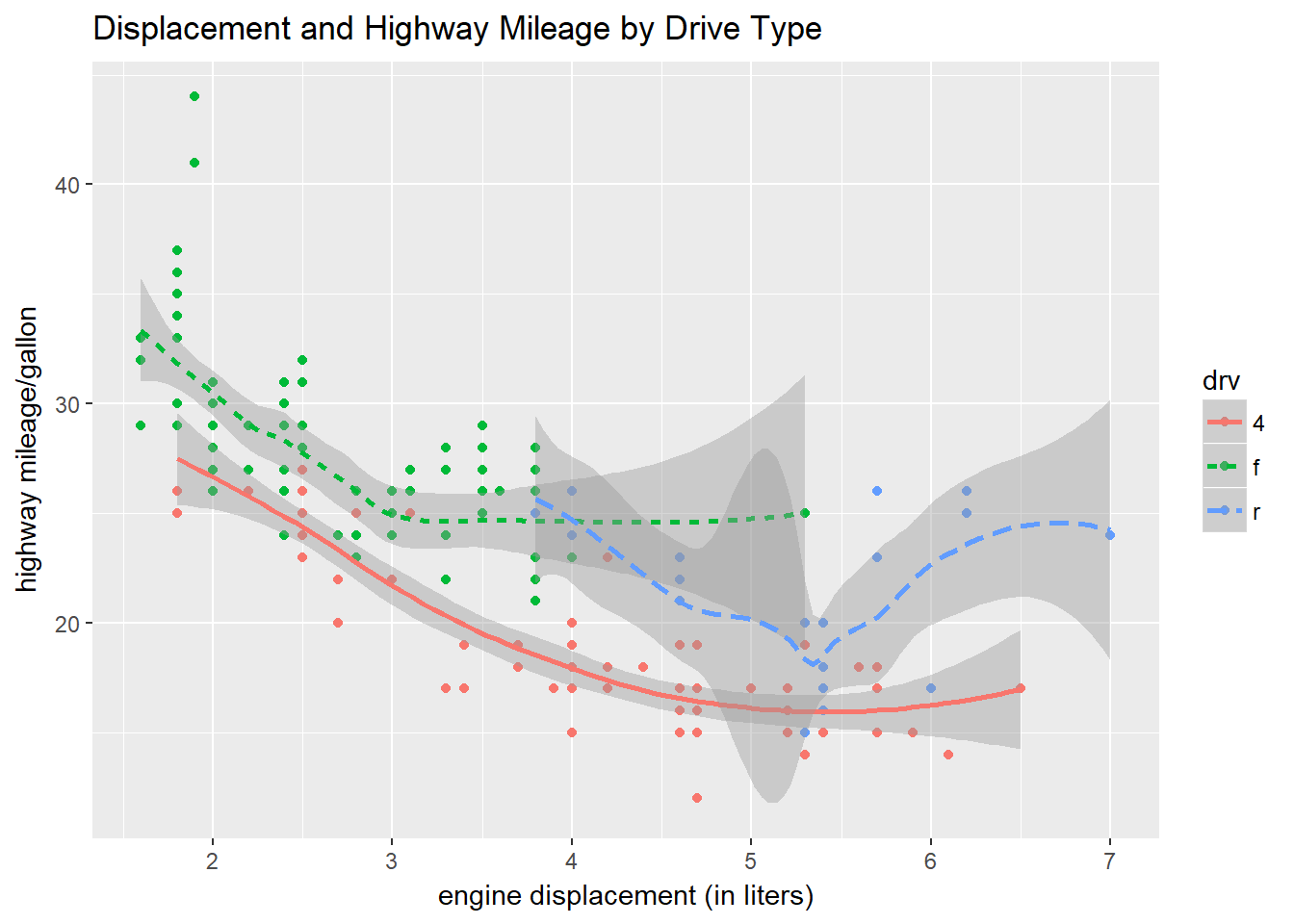We can take advantage of a feature of ggplot to create the same graph a slightly different way.

You notice in the above code chunk there is some duplication in the geom_point and geom_smooth arguments. It is generally a good practice to avoid duplication if possible. If we move the mapping arguments color = drv and linetype = drv to aes in ggplot itself we can leave the geom_point and geom_smooth arguments empty. This is a useful trick if you are changing variables around. You have to adjust them only once rather than in each argument where they may have lived before. In R-speak, we have moved local mappings to global mappings.

ggplot(data = mpg, mapping = aes(x = displ, y = hwy, color = drv, linetype = drv)) +
geom_point() + geom_smooth() +
ggtitle("Displacement and Highway Mileage by Drive Type") +
labs(x = "engine displacement (in liters)", y = "highway mileage/gallon")
## `geom_smooth()` using method = 'loess'By doing this, we gain the ability to use different mappings with different aesthetics in the same chart. In this example, we are going to depict fuel type fl in the individual data points, and change the geometric smoothing of the regression line using span.

What do you suppose those two green diesel outliers are about?

ggplot(data = mpg, mapping = aes(x = displ, y = hwy, color = drv, linetype = drv)) +
geom_point(aes(shape = fl)) + geom_smooth(span = 0.6) +
ggtitle("Displacement and Highway Mileage by Drive Type and Fuel") +
labs(x = "engine displacement (in liters)", y = "highway mileage/gallon")
## `geom_smooth()` using method = 'loess'